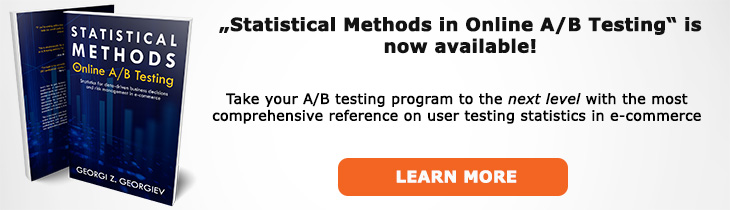# What does "Percent Change" mean?

Definition of Percent Change in the context of A/B testing (online controlled experiments).

## What is Percent Change?

Aliases: lift, relative change, relative difference

Percent change, a.k.a. percent lift, relative change or relative difference, is the difference between two variables of interest relative to one of the variables. The percentage lift on some Key Performance Indicator (KPI) is often what is reported as the outcome of an A/B test, but often it is done using incorrect methods (see below).

The relative change is calculated as the absolute difference between two variables divided by one of them, usually that of the control group. To get a percent change one needs to multiply the relative change by 1000. Relative change is often written as δRel(B,A) or δRel = (μ2 - μ1)/μ1, equivalently δabs = (p2 - p1)/p1 for proportions. For example, if the proportion in group B is 0.05 and the proportion in group A is 0.04, then δRel(B,A) = (0.05 - 0.04) / 0.04 = 0.01 / 0.04 = 0.25. Multiply by 100 will give us 25% percent change.

Since most of the time in A/B testing the absolute differences are very small and often not very informative unless one also communicates the baseline, we prefer to report the relative difference (percent change, % lift) since calculating business effect is more straightforward when doing so. Since most traditional significance tests and confidence intervals are developed to address the question in terms of absolute difference, it is no wonder that many CRO practitioners get this wrong and fail to use a method that appropriately takes into account the added variance introduced by dividing by one of the variables.

## Related A/B Testing terms

Absolute Difference

## Articles on Percent Change

Like this glossary entry? For an in-depth and comprehensive reading on A/B testing stats, check out the book "Statistical Methods in Online A/B Testing" by the author of this glossary, Georgi Georgiev.## Glossary Index by Letter

Select a letter to see all A/B testing terms starting with that letter or visit the Glossary homepage to see all.